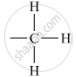# Give the Name and Structural Formula of an Alkyl Group. - Science

Give the name and structural formula of an alkyl group.

#### Solution

The general formula of an alkyl group is CnH2n+1 where n is an integer. A methyl group (-CH3) is a derivative of methane (CH4) with the structural formula as shown below:Is there an error in this question or solution?

#### APPEARS IN

Lakhmir Singh Class 10 Chemistry (Science)
Chapter 4 Carbon And Its Compounds
Q 12 | Page 221